# Boolean Algebra

May 19 • General • 5785 Views • 3 Comments on Boolean AlgebraNotes on Boolean Algebra

In Mathematics, Boolean algebra is part of the algebra. It also includes variables and constants, the values of the variables which comes out after the calculations and using all the logic are the truth values either TRUE or FALSE. i.e. the binary values 0 or 1

Boolean algebra is widely used in computers.

Instead of other algebra in which the values are numbers which comes after doing various operations like addition, subtraction, multiplication division etc. The main operations used in Boolean algebra are conjunction which is denoted by ^ other operators are disjunction denoted by ᵥ   and negation denoted by ¬.

Basic Operations :

1. AND (conjunction) denoted by ^

2. OR (disjunction) denoted by ᵥ

3. NOT (negation) denoted by ¬

If we combine these operations with basic mathematical operations  then it can be reprsented as :

x^y = xy

xᵥy = x+y – xy

¬x = 1-x

The values of above expression can be explained with the help of truth tables. They are explained as under :

1. x^y

2. xᵥy

 x y x^y x ᵥy 0 0 0 0 1 0 0 1 0 1 0 1 1 1 1 1

3. xᵥy

 x ¬x 0 1 1 0

Some laws i.e. the MONOTONE LAWS used here are :

1. Associativity

x∨(yz) = (xy)∨z

x∧(yz) = (xy)∧z

2. Commutativity

xy = yx

xy = yx

3. Distributivity

x∧(yz) = )=(x∧y)∨(x∧z)

4. Identitivity

x∨0 = x

x∧1=x

x∧0=0

Example

1.  Verify that the following operations are commutative

a. AND  b. OR c. XOR

a. AND

A . B = B . A ( therefore AND operation is commutative)

b. OR

A + B = B + A (therefore OR operation is commutative)

2.Derive the Boolean expression for the logic circuit shown below: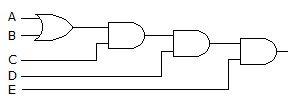A.B.C.D.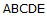3. From the Truth table below, Determine the boolean expression :

From the truth table below, determine the standard SOP expression.

 Input Output A B C X 0 0 0 0 0 0 1 1 0 1 0 0 0 1 1 1 1 0 0 0 1 0 1 0 1 1 0 1 1 1 1 0

 A.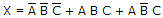B.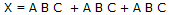C.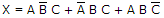D.### 3 Responses to Boolean Algebra

1. suriya prakash says:

strong in basics is essential to reach any level of depth knowledge. this article explains the basics of boolean algebra very well.

2. Rachita Mishra says:

This article is about the subarea of mathematics. For the related algebraic structures, see Boolean algebra (structure) and Boolean ring. In mathematics and mathematical logic, Boolean algebra is the subarea of algebra in which the values of the variables are the truth values true and false, usually denoted 1 and 0 respectively.

3. vishrut patel says:

A complete notes on boolean algebra with its operations,laws and truth table is mentioned i this article.It can be very helpfull…